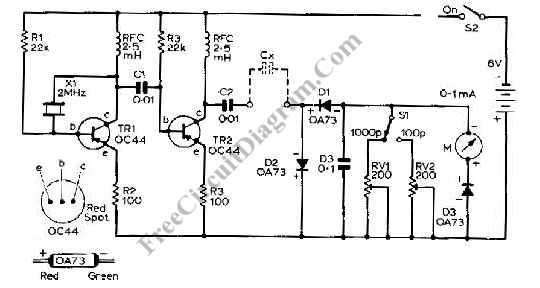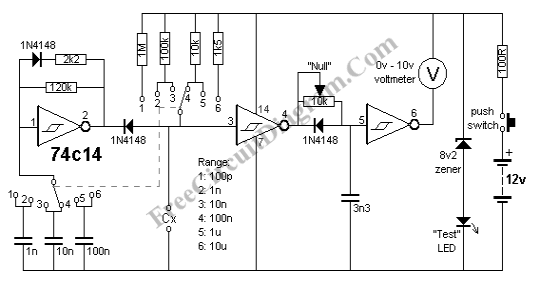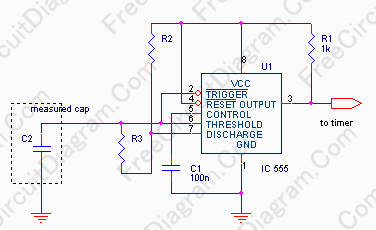## Capacitance Meter Circuit Using TransistorsThis capacitance meter circuit is similar with previous meter circuit, but it uses transistors rather than logic gates. Here is the schematic diagram: You can see the circuit uses pnp transistor, you can use almost any pnp transistors with frequency bandwidth og 100MHz and collecttor current handling capability of 100 mA to replace the transistor if you can find the […]

## Capacitance MeterCapacitance meter can be very helpful in identifying old components where its mark has been erased or became unreadable. Such instrument can be quite expensive, but fortunately we can build it easily with much lower cost. The capacitance meter described here can measure any capacitor between 1pF and 10uF. Here is the schematic diagram of the circuit: You can just […]